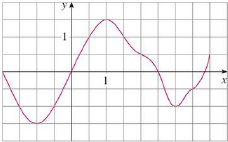Chapter 5.2, Problem 6E### Single Variable Calculus: Early Tr...

8th Edition
James Stewart
ISBN: 9781305270343

#### Solutions

Chapter
Section### Single Variable Calculus: Early Tr...

8th Edition
James Stewart
ISBN: 9781305270343
Textbook Problem

# The graph of g is shown. Estimate ∫ − 2 4 g ( x ) d x with six subintervals using (a) right endpoints, (b) left endpoints, and (c) midpoints.(a)

To determine

To estimate: The value of the integral 24g(x)dx by using the right end points.

Explanation

The expression of the Riemann sum using the right end points is shown below:

Rn=i=1nf(xi)Δx=Δx(f(x1)++f(xn)) (1)

Apply the condition for Riemann sum as follows:

Δx=ban (2)

Here, width of the subinterval is Δx, the upper limit is b, the lower limit is a, the number of subinterval is n, and the right endpoint of (xi1,xi) is xi1.

Find the width of the subinterval (Δx) using Equation (2) as shown below.

Consider the number of subintervals as 6.

Substitute 6 for n, 4 for b and -2 for a in Equation (2).

Δx=4(2)6=1

Calculate the value of Riemann sum using the right end points.

Refer to the graph of the function g.

The right end points of the intervals are -1, 0, 1, 2, 3, and 4.

Substitute 6 for n, 1 for Δx, -1 for x1, 0 for x2, 1 for x3, 2 for x4, 3 for x5, and 4 for x6 in Equation (1) as shown below

(b)

To determine

To estimate: The value of the integral 24g(x)dx using the left end points.

(c)

To determine

To estimate: The value of the integral 24g(x)dx by using the midpoints.

### Still sussing out bartleby?

Check out a sample textbook solution.

See a sample solution

#### The Solution to Your Study Problems

Bartleby provides explanations to thousands of textbook problems written by our experts, many with advanced degrees!

Get Started

#### In Exercises 21-24, find the distance between the given points. 22. (1, 0) and (4, 4)

Applied Calculus for the Managerial, Life, and Social Sciences: A Brief Approach

#### If f(x)=0xx2sin(t2)dt, find f(x).

Single Variable Calculus: Early Transcendentals

#### The polar form for the graph at the right is:

Study Guide for Stewart's Single Variable Calculus: Early Transcendentals, 8th